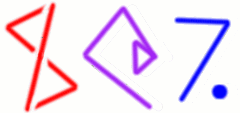# Arithmetic with Continued FractionsLength: 60 minutes

## Description

Multiprecision arithmetic algorithms usually represent real numbers as decimals, or perhaps as their base-2n analogues. But this representation has some puzzling properties. For example, there is no exact representation of even as simple a number as one-third. Continued fractions are a practical but little-known alternative.

Continued fractions are a representation of the real numbers that are in many ways more mathematically natural than the usual decimal or binary representations. All rational numbers have simple representations, and so do many irrational numbers, such as sqrt(2) and e1. One reason that continued fractions are not often used, however, is that it's not clear how to involve them in basic operations like addition and multiplication. This was an unsolved problem until 1972, when Bill Gosper found practical algorithms for continued fraction arithmetic.

In this talk, I explain what continued fractions are and why they are interesting, how to represent them in computer programs, and how to calculate with them.